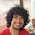### Horse Race

Source: http://www.qbyte.org/puzzles/puzzle14.html

Problem: (An interesting counting exercise)
In how many ways, counting ties, can eight horses cross the finishing line?
(For example, two horses, A and B, can finish in three ways: A wins, B wins, A and B tie.)

Update(21/01/10): (simple combinatorics problem)
Solution: Solution posted by Nikhil Garg (Sophomore, IIT Delhi) in comments!!
Update(06/02/10): Interesting solution posted by Aman too.

1.General solution assuming n horses.

Let all horses which reach at same time (have a tie ) belong to a group. Finally let there are k groups of horses. ( 1<=k<=n) . We can partition n horses in k such sets in S(n,k) ways. Now once we have k groups , we can arrange their order in k! ways.
So number of ways in which k groups are there is : S(n,k) * k !

summation over all k -> S(n,k) * k!

2.Thanx. Elegant and simple solution to a very simple problem. :) Nice

3.eejee. however for the readers, you should bother to specify that S(n,k) are the stirling numbers of the second kind, or rather the obv recursive relation is S(n,k)= k*S(n-1,k) + S(n-1,k-1) or whtever:P

4.Another solution:

The number of ways in which k ranks can be allotted to n horses such that each rank is allotted at least once is :

coeff. of x^n in
n!*(x + x^2/2! + x^3/3!......)^k
= n!*(e^x -1)^k

summing the above over all k and collecting the coefficients gives the answer.

5.Ramdas is referring to "Stirling numbers of second kind"
Thanx :)

@Aman.. How? Am I missing something? :(

6.I accept, it wasn't so trivial, I should have explained it before.

Here is the explanation:

Suppose the horses are divided into k groups with x1 in 1st, x2 in 2nd and so on.
Then the number of permutations with this particular grouping is n!/(x1!*x2!.....*xk!)

The above expression essentially gives the sum of all such permutations with different values of x1,x2...xk such that x1 + x2 +....xk = n (i.e. all possible different groupings)

We can extend each of the expressions (x + x^2/2! .....)
upto infinity as none of the higher terms contribute to the desired coeff. of x^n.

7.Awesome.. good one.. Thanx..

8.I got a recursive solution which I wasn't able to convert in closed form. The logic is this -
Let N(i) be the solution for i horses
Then N(n) = summation(nCi*N(n-i)) for i in {1,...,n}
It can be explained as follows - pick only one horse for 1st rank in nC1 ways and multiply it by N(n-1) else pick exactly two in nC2 and multiply by N(n-2) and so on.

I don't know what Stirling numbers are but since those are themselves recursive, I suppose there is no closed form solution to this.

9.Looks correct to me :)

10.Win Bets Consistently It Teaching Horse Race Betters The Correct Procedures To Win Bets On High-odds Horses Consistently And To Enjoy The Races. It Also Teaches Betting Strategies